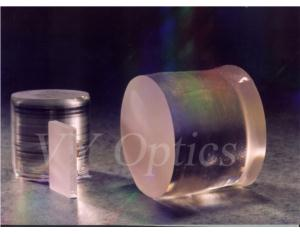.

#ok
xMember ID or Email
Join Free
Home >Members & Products > Thick CLevel Car Carpet## Member Details

Changchun,Jilin,China
• Product Details
• Company Profile

Lithium Niobate(LiNbO3) Crystal lens is widely used as frequency doublers for wavelength > 1 mm and optical parametric oscillators (OPOs) pumped at 1064 nm as well as quasi-phase-matched (QPM) devices. Due to its large Electro-Optic (E-O) and Acousto-Optic (A-O) coefficients. We manufacture LiNbO3 crystal lens professionally. Basic Properties of optical LiNbO3 crystal lens as follow: Crystal Structure:Trigonal, point group 3m Lattice Parameters:a=b=5.148 , c=13.863 Density:4.64 g/cm3 Melting Point:1250 degrees Curie Point:1142+/- degrees Mohs Hardness:55 Dielectric Constant :e11/e0 = 85; e33/e0 = 29.5 Thermal Conductivity:38 W/m egrees at 25 egrees Thermal Expansion Coefficient:a1=a2 =2x10-6 egrees, a3=2.2x10-6/ degrees at 25degrees Piezoelectric Strain Constant:d22 = 2.04 x 10-11 C/N, d33 = 19.22 x 10-11 C/N Elastic Stiffness Constant:CE11 = 2.04 x 1011 N/m2, CE33 = 2.46 x 1011 N/m2

Lithium Niobate(LiNbO3) Crystal lens is widely used as frequency doublers for wavelength > 1 mm and optical parametric oscillators (OPOs) pumped at 1064 nm as well as quasi-phase-matched (QPM) devices. Due to its large Electro-Optic (E-O) and Acousto-Optic (A-O) coefficients.

We manufacture LiNbO3 crystal lens professionally.
Basic Properties of optical LiNbO3 crystal lens as follow:
Crystal Structure:Trigonal, point group 3m
Lattice Parameters:a=b=5.148 , c=13.863
Density:4.64 g/cm3
Melting Point:1250 degrees
Curie Point:1142+/- degrees
Mohs Hardness:55
Dielectric Constant :e11/e0 = 85; e33/e0 = 29.5
Thermal Conductivity:38 W/m egrees at 25 egrees
Thermal Expansion Coefficient:a1=a2 =2x10-6 egrees, a3=2.2x10-6/ degrees at 25degrees
Piezoelectric Strain Constant:d22 = 2.04 x 10-11 C/N, d33 = 19.22 x 10-11 C/N
Elastic Stiffness Constant:CE11 = 2.04 x 1011 N/m2, CE33 = 2.46 x 1011 N/m2

# Send Inquiry to This Supplier

• Title
• From*
• toMary Ju
• Message*
• Quantity
• Unit
• Verification Code*